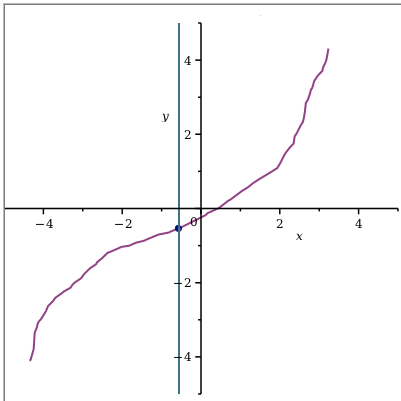Taylor's Theorem - Maple Help

Home : Support : Online Help : Math Apps : Calculus : Differential : Taylor's Theorem

Taylor's Theorem

 Main Concept The Taylor Series Approximation of order $n$ to the ($n$-times differentiable) function $f\left(x\right)$ is given by:   .    Taylor's Theorem essentially states that ${f}_{n}$ is the best possible degree $n$ polynomial approximation to $f$ about the point $a$. Specifically, the difference, $f\left(x\right)-{f}_{n}\left(x\right)$ can be written in the form:   $f\left(x\right)-{f}_{n}\left(x\right)={h}_{n}\left(x\right)\cdot {\left(x-a\right)}^{n}$, where $\underset{x\to a}{{lim}}\phantom{\rule[-0.0ex]{0.4em}{0.0ex}}{h}_{n}\left(x\right)=0$.   The special case of Taylor Series in which  is known as the Maclaurin Series.

Pick the function you want to approximate and adjust the sliders to modify the settings.sin(x)cos(x)x^3 sinh(x)cosh(x)More MathApps